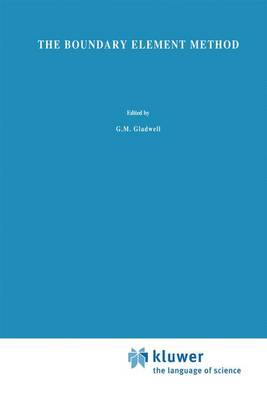•# The Boundary Element Method - Solid Mechanics and Its Applications 27 (Hardback)

(author)
£199.99
Hardback 230 Pages
Published: 30/11/1993
• We can order this from the publisher

UK delivery within 3-4 weeks

The Boundary Element Method is a simple, efficient and cost effective computational technique which provides numerical solutions - for objects of any shap- for a wide range of scientific and engineering problems. In dealing with the development of the mathematics of the Boundary Element Method the aim has been at every stage, only to present new material when sufficient experience and practice of simpler material has been gained. Since the usual background of many readers will be of differential equations, the connection of differential equations with integral equations is explained in Chapter 1, together with analytical and numerical methods of solution. This information on integral equations provides a base for the work of subsequent chapters. The mathematical formulation of boundary integral equations for potential problems - derived from the more familiar Laplace partial differential equation which governs many important physical problems - is set out in Chapter 2. It should be noted here that this initial formulation of the boundary integral equations reduces the dimensionality of the problem. In the key Chapter 3, the essentials of the Boundary Element Method are presented. This first presentation of the Boundary Element Method is in its simplest and most approachable form - two dimensional, with the shape of the boundary approximated by straight lines and the functions approximated by constants over each of the straight lines.

Publisher: Springer
ISBN: 9780792325802
Number of pages: 230
Weight: 1150 g
Dimensions: 235 x 155 x 14 mm
Edition: 1994 ed.